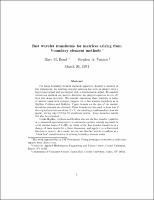## Fast Wavelet Transforms for Matrices Arising from Boundary Element Methods##### Abstract
(The following contains mathematical formulae and symbols that may become distorted in ASCII text.) For many boundary element methods applied to Laplace's equation in two dimensions, the resulting integral equation has both an integral with a logarithmic kernel and an integral with a discontinuous kernel. If standard collocation methods are used to discretize the integral equation we are left with two dense matrices. We consider expressing these matrices in terms of wavelet bases with compact support via a fast wavelet transform as in Beylkin, Coifman, and Rokhlin. Upper bounds on the size of the wavelet transform elements are obtained. These bounds are then used to show that if the original matrices are of the size N x N, the resulting transformed matrices are sparse, having only O(N log N) significant entries. Some numerical results will also be presented. Unlike Beylkin, Coifman and Rokhlin who use the fast wavelet transform as a numerical approximation to a continuous operator already expressed in a full wavelet basis of L2(IR), we think of the fast wavelet transform as a change of basis matrix for a finite dimension, and apply it to a discretized function or matrix. As a result, we can use this fast wavelet transform as a "black box" transformation in existing boundary element codes.
1994-03
##### Publisher
Cornell University
theory center
##### Previously Published As
http://techreports.library.cornell.edu:8081/Dienst/UI/1.0/Display/cul.tc/94-174
technical report